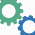Thursday, April 7, 2011

Ruby Koans: Greed Dice Game

I was working on the Greed dice game in the Ruby Koans. My goal was to come up with an algorithm that was elegant, readable, and rubyesque. This is what I came up with:

```def score(dice)
score = 0
points = Hash.new(0)
points = 100
points = 50

counts = Hash.new(0)
dice.each { |number| counts[number] += 1 }

counts.each do |number, count|
if count >= 3
if number == 1
score += 1000
else
score += (number * 100)
end
count = count - 3
end

score += points[number] * count
end

score
end

```

If you're not familiar with the ruby koans, here's the link to the path of enlightenment: Ruby koans

1.You can use `inject` to load the hash and a case statement to iterate through the possible die sides:

def score(dice)
tally = 0

return tally if dice.length == 0

hash = dice.inject(Hash.new(0)) { |h,v| h[v] += 1; h }

(1..6).collect do |roll|
case roll
when 5
tally += (hash[roll] / 3) * 500 + (hash[roll] % 3) * 50
when 1
tally += (hash[roll] / 3) * 1000 + (hash[roll] % 3) * 100
else
tally += (hash[roll] / 3) * roll * 100
end
end

ap "dice = #{dice}, " + "hash = #{hash}, " + "tally = #{tally}"

tally
end

2.3.Hi dear, I like your post so much, and it is very good. Hope that we can communicate with each other. I want to introduce an aso services, I try it and I feel it is so good to us beginners.

4.This was really interesting info in this blog that to very happy for the nice technology in this blog. best live gambling

5.